## BYROW Function in Google Sheets – LAMBDA Helper Function

The BYROW function in Google Sheets operates on an array or range and returns a new column array, created by grouping each row to a single value.

The value for each row is obtained by applying a lambda function on that row.

For example, this BYROW function calculates the average score of all three rows in the input array:

`=BYROW(A2:D4,LAMBDA(row, AVERAGE(row)))`

Which looks like this in our Google Sheet: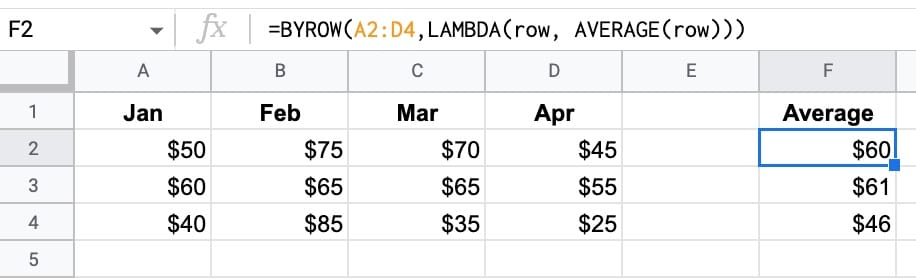(Of course, you could use three separate AVERAGE functions to perform this calculation.)

But the important thing here is how the BYROW operates. It’s much more like programming.

We pass an array of data to the BYROW function.

It then passes each row into a lamba function to calculate a single value for that row (the average in this example). The BYROW formula returns these values in a column array, with the same number of rows as the original input array.

🔗 Get this example and others in the template at the bottom of this article.

## MAKEARRAY Function in Google Sheets – LAMBDA Helper Function

The MAKEARRAY function in Google Sheets generates an array of a specified size, with each value calculated by a custom lambda function.

The Lamba function has access to the row and column indices for each value.

Let’s see a simple example:

`=MAKEARRAY(5,3,LAMBDA(row, col, row + col))`

This generates an array with 5 rows and 3 columns.

The value of each element in the row is the sum of the row position and the column position, within the array.

So the first value is 2 (row 1 + column 1) and the final value is 8 (row 5 + column 3). The indices are in relation to the position within the array, not the position within the Google Sheet.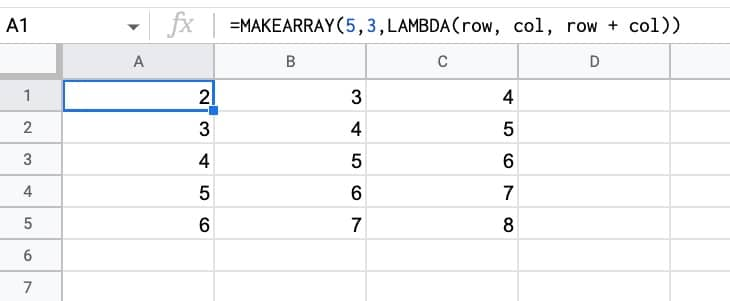🔗 Get this example and others in the template at the bottom of this article.

## SCAN Function in Google Sheets – LAMBDA Helper Function

The SCAN function in Google Sheets scans an array and applies a LAMBDA function to each value, moving row by row. The output is an array of intermediate values obtained at each step.

It’s hard to understand without seeing an example, so let’s do that.

The most straightforward SCAN formula is to create a running total calculation on a column of numbers.

The formula is:

`=SCAN(0,A2:A11,LAMBDA(runningTotal,currentValue,runningTotal + currentValue))`

Which produces this array output: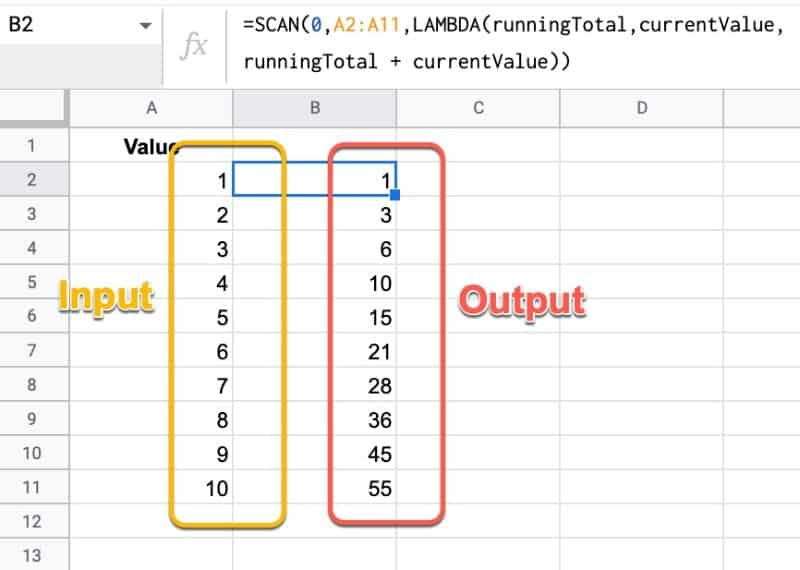The lambda simply adds together the current running total and the new value, then returns the answer to the output array.

When all of the values from the input array have been considered, the output array is returned.

🔗 Get this example and others in the template at the bottom of this article.

## REDUCE Function in Google Sheets – LAMBDA Helper Function

The REDUCE function in Google Sheets turns an array input into a single value, by applying a custom LAMBDA function to each value of the input array.

It reduces an array down to a single value.

It’s best to understand through a simple example: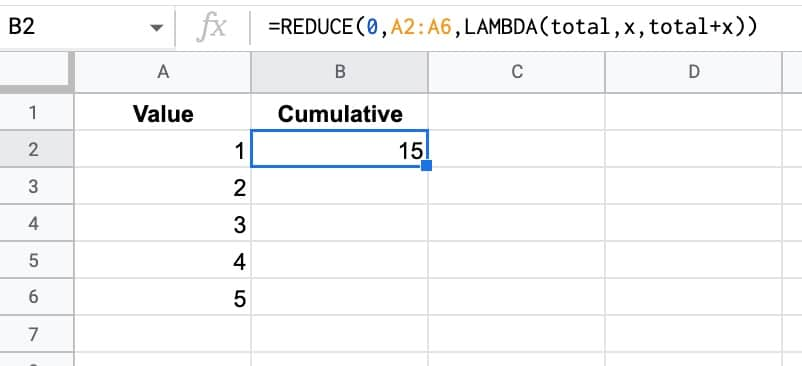The formula in this example is:

`=REDUCE(0,A2:A6,LAMBDA(total,x,total+x))`

The REDUCE function takes a starting value (0 in this example), an input array, and then a custom LAMBDA function.

The formula applies the custom LAMBDA function to each value in the array. In this case, it takes the current total value and adds the next value in the array to it to make a new total. This new total is passed on to the next iteration.

So this REDUCE formula calculates the cumulative total of the 5 numbers.

(And yes, this formula also gets you there:

`=SUM(A2:A6)`

This REDUCE was deliberately simple to show you how it works.)

🔗 Get this example and others in the template at the bottom of this article.

## MAP Function in Google Sheets – LAMBDA Helper Function

The MAP function in Google Sheets creates an array of data from an input range, where each value is “mapped” to a new value based on a custom LAMBDA function.

MAP takes an input range of data from your Sheet, then performs an operation on each value of that range, and puts the results into an output array of the same dimensions as the original array.

Let’s see a silly example to understand how the MAP function works.

We’re going to transform an array of data into thumbs up for values 5 and over, and skull and crossbones for values less than 5. Stay with me here 😉

Here’s the input array: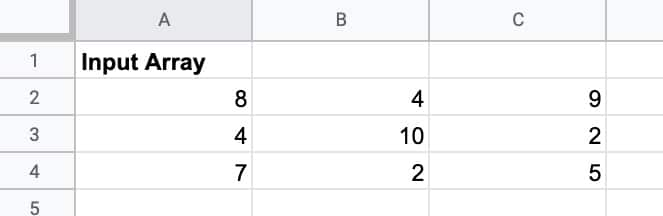To which we apply this MAP formula:

`=MAP(A2:C4, LAMBDA(value, IF(value >= 5 , "👍" , "☠️" )))`

MAP passes each value to the LAMBDA, which uses a regular IF function to test if the value is greater than or equal to 5, and output a thumbs up or skull.

To give an output array of: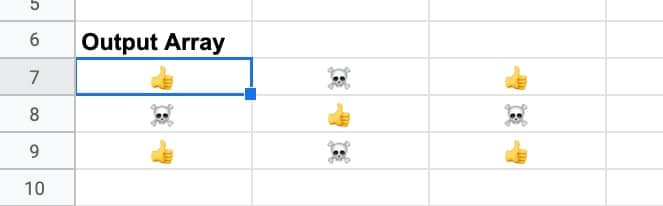If I highlight the values, you can see how MAP evaluates each value against the test, and transforms it into a thumbs up or a skull and crossbones.

The 9 has mapped to a thumbs up, and the 2 has mapped to a skull and crossbones.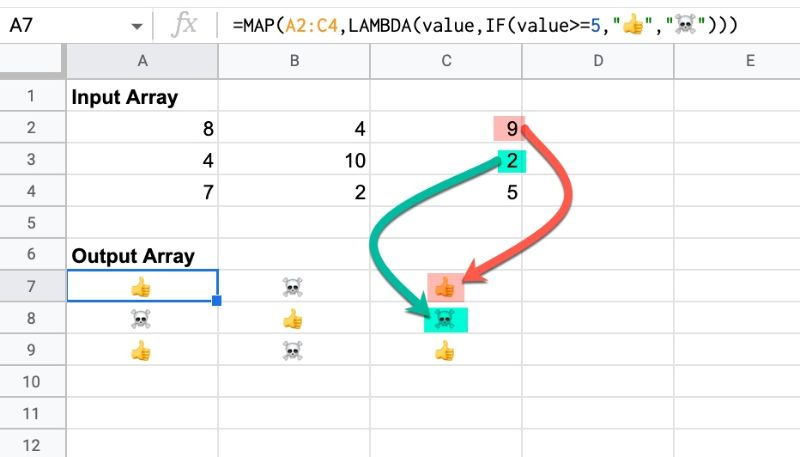Ok, so this isn’t a particularly useful example, but it’s a nice illustration of how the MAP function works.

There are some more practical examples further down this post.

🔗 Get this example and others in the template at the bottom of this article.